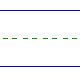Name: ______________________________________________ Date: _____________

Subtraction

Instructions: Subtract.

 6 - 6 =9 - 1 =2 - 1 =7 - 1 =2 - 0 =9 - 0 =6 - 4 =5 - 1 =9 - 3 =9 - 7 =8 - 6 =4 - 2 =4 - 3 =3 - 3 =7 - 6 =9 - 2 =8 - 4 =5 - 2 =